AP Calculus AB : Integrals

Example Questions

Example Question #3 : Antiderivatives Following Directly From Derivatives Of Basic Functions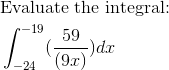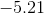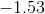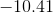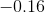Explanation: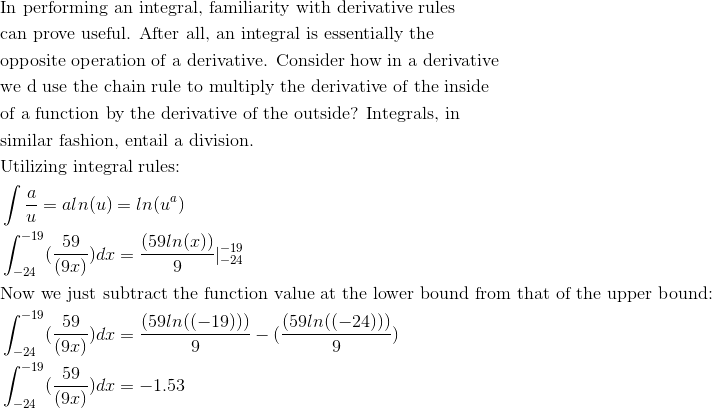Example Question #4 : Antiderivatives Following Directly From Derivatives Of Basic Functions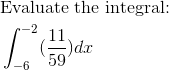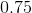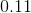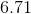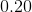Explanation: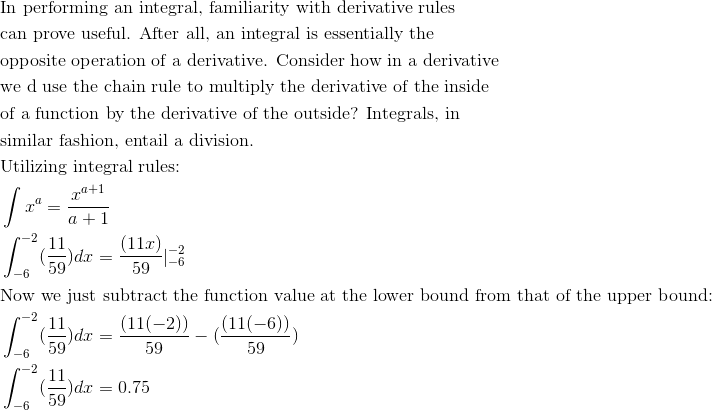Example Question #5 : Antiderivatives Following Directly From Derivatives Of Basic Functions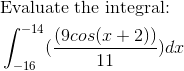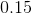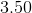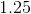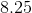Explanation:Example Question #11 : Techniques Of Antidifferentiation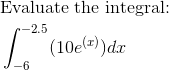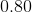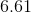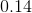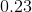Explanation: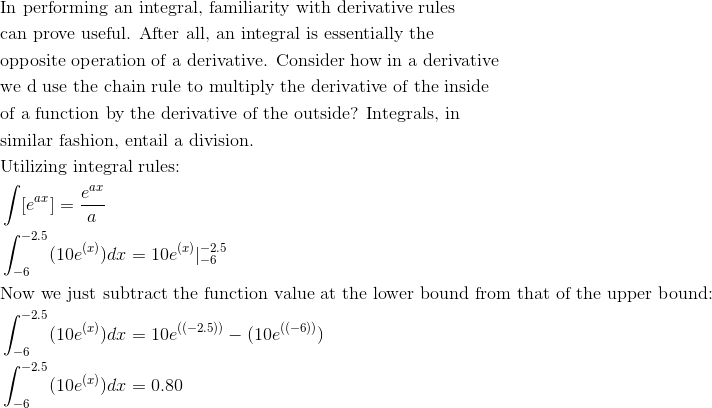Example Question #12 : Techniques Of Antidifferentiation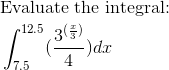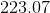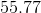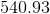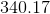Explanation: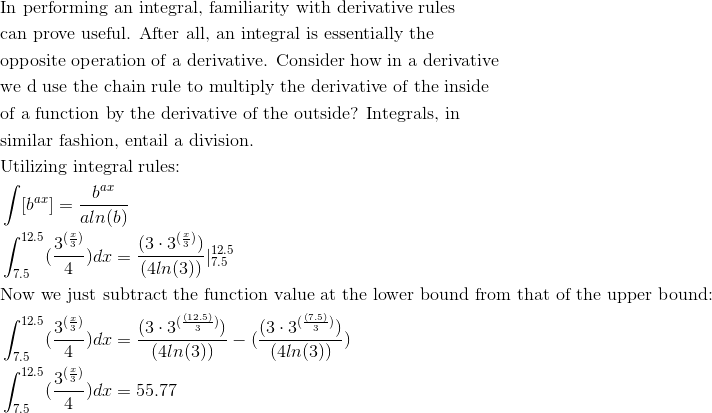Example Question #13 : Techniques Of Antidifferentiation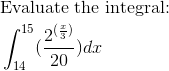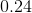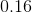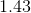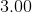Explanation: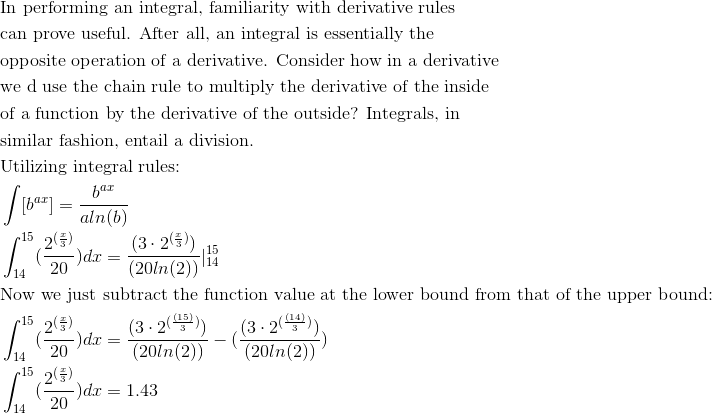Example Question #14 : Techniques Of Antidifferentiation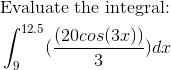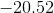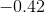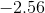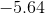Explanation: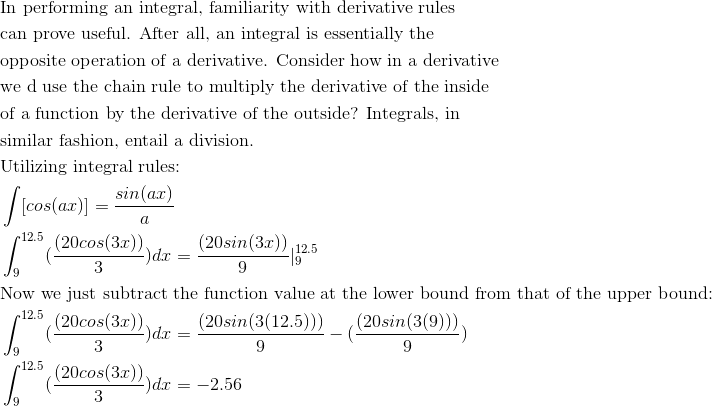Example Question #81 : Integrals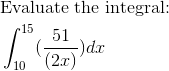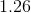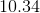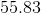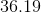Explanation: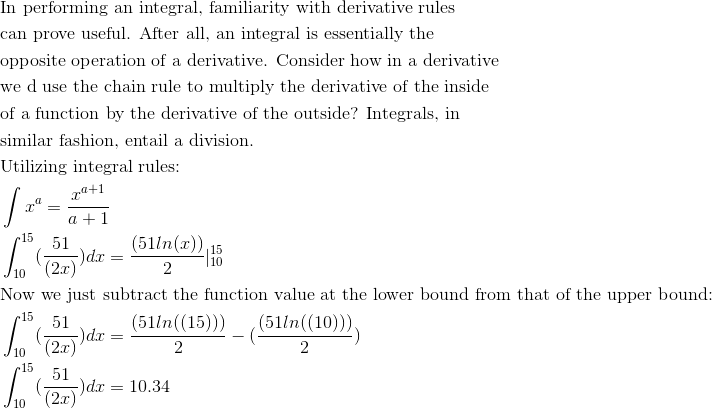Example Question #21 : Techniques Of Antidifferentiation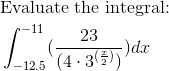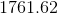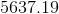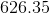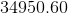Explanation: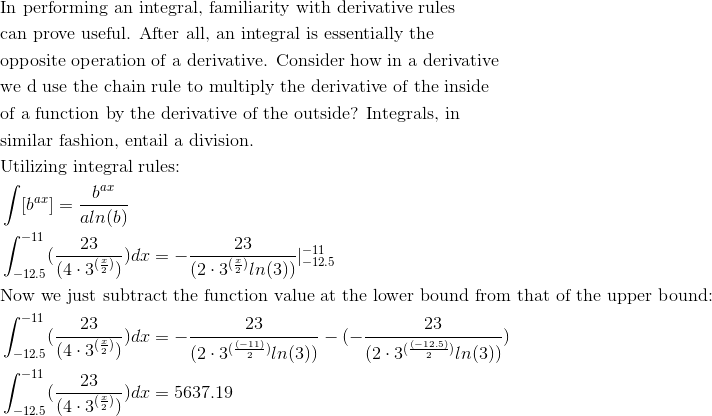Example Question #22 : Techniques Of Antidifferentiation

Given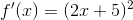, find the general form for the antiderivative.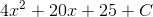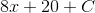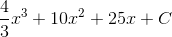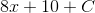Explanation:

To answer this, we will need to FOIL our function first.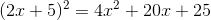Now can find the antiderivatives of each of these three summands using the power rule.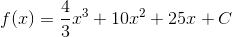(Don't forget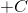)!